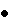Software development is inherently risky.  According to Rochelle Gardner [ROC95], about one-fourth of projects greater than 5,000 function points are canceled.  The Standish Group conducted a survey of 365 organizations representing 8,380 applications.  According to this survey, the following represents the percent of achieved functions in troubled/challenged projects [JON95]:Less that 25% of functions installed - 5% of the projects25% - 49% of functions installed - 27% of the projects50% - 74% of the functions installed - 22% of the projects75% - 99% of the functions installed - 39% of the projects100% of the functions installed - 7% of the projectsThe tremendous advances in hardware technologies have enabled similar advances in the tools available to software developers.  The result is larger and more complicated systems that have become much more critical to our every day lives.  This rapid change makes systems more complex and therefore more error prone.  When the systems do not work properly, the costs can be very significant -- both in dollars and in human lives.In defect management, we define risk as the potential for a problem.  The problem could be the result of a defect, a failure, or it could be any situation or event that would increase the cost of the system, delay its benefits, or reduce its benefits.  A critical risk is a risk that if it becomes a problem, then successful delivery and/or operation of the system is in jeopardy.

Risk is inherent in all development projects. The following example illustrates why this is true. Let's assume that a typical project has 4 critical risks that each have a relatively low probability of occurring.  The probability of each risk not being a problem is fairly high as shown below.

Mathematics of Risk

Success = No Critical Risk Becomes a Problem

Example:     4 Critical Risks

 Probability that the target date will not be impacted = 80% Probability that requirements will be met = 90% Probability that the user will accept the system = 80% Probability that cost will not be overrun  = 70% *Probability of Success = 40%*The Probability of Success is the probability that no critical risk will become a problem.  In the above example, the calculation is: (.8)(.9)(.8)(.7) = (.4032).

There are usually many critical risks on projects.  Even with low individual risks, the combined probability of at least one critical risk occurring is very high.  In this example, success can be equated to no critical risks.  But the probability of no critical risks occurring is the product of the probability of each risk not occurring (.8*.9*.8*.7 = .4).  The project thus has less than a 50% probability of success and is not likely to succeed.  This simplified example provides insight into why so many software development projects are canceled.  There are simply too many critical risks and the combined probability that one or more of the risks will become a problem is very high.

To mitigate this, developers require strategies that are designed to reduce:The number of critical risks.The probability that a risk will become a problem.The impact of a problem if it does occur.